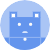search
Search
menu search toc more_vert
Guest 0reps
Thanks for the thanks!
close
chevron_left Handling Missing Values
Cancel
Post
account_circle
Profile
exit_to_app
Sign out
help Ask a question
search
keyboard_voice
close
Searching Tips
Search for a recipe:
"Creating a table in MySQL"
Search for an API documentation: "@append"
Search for code: "!dataframe"
Apply a tag filter: "#python"
Useful Shortcuts
/ to open search panel
Esc to close search panel
to navigate between search results
d to clear all current filters
Enter to expand content previewDoc SearchCode Search BetaSORRY NOTHING FOUND!
mic
Start speaking...Voice search is only supported in Safari and Chrome.
Shrink
Navigate to
A
A
brightness_medium
share
arrow_backShareTwitterFacebook
chevron_left Handling Missing Values
0
thumb_down
0
chat_bubble_outline
0
auto_stories new
settings

# Pandas DataFrame | dropna method

Pandas
chevron_right
Documentation
chevron_right
DataFrame
chevron_right
Handling Missing Values
schedule Jul 1, 2022
Last updated
local_offer PythonPandas
Tags

Pandas `DataFrame.dropna(~)` method removes rows or columns with missing values.

# Parameters

1. `axis`link | `int` or `string` | `optional`

Whether or not to remove rows or columns with missing values:

Axis

Description

Scans through each row, and if a missing value exists, drop the row.

`0` or `"index"`

Scans through each column, and if a missing value exists, drop the column.

`1` or `"columns"`

By default, `axis=0`.

2. `how`link | `string` | `optional`

The criteria by which to remove a row/column:

How

Description

`"any"`

If the row or column consists of at least one missing value, then remove it.

`"all"`

If the row or column consists of all missing values, then remove it.

By default, `how="any"`.

3. `thresh` | `int` | `optional`

The number of non-`NaN` a row/column must at least contain to not be dropped. For instance, if `thresh=2`, then

• a column with 1 non-missing value will be dropped.

• a column with 2 non-missing values will be kept.

• a column with 3 non-missing values will be kept.

By default, no minimum is set.

4. `subset`link | `array-like` of `strings` | `optional`

The columns to check for missing values when scans are performed row-wise (when `axis=0`). By default, all columns are considered. Consult examples below for clarification.

5. `inplace`link | `boolean` | `optional`

• If `True`, then the method will directly modify the source DataFrame instead of creating a new DataFrame.

• If `False`, then a new DataFrame will be created and returned.

By default, `inplace=False`.

# Return Value

A `DataFrame` with rows or columns that contain missing values removed according to the provided parameters.

# Examples

Consider the following DataFrame:

``` df = pd.DataFrame({"A":[pd.np.NaN,2],"B":[3,4],"C":[5,6]})df    A    B  C0  NaN  3  51  2.0  4  6 ```

## Removing rows with missing values

To remove rows with missing value(s):

``` df.dropna()   # or axis=0 or axis="index"    A    B  C1  2.0  4  6 ```

Notice how the first row (i.e. `index=0`) was removed since it contained a missing value.

## Removing columns with missing values

To remove columns with missing value(s):

``` df.dropna(axis="columns")   # or axis=1    B  C0  3  51  4  6 ```

Notice how column `A` was removed since it contained a missing value.

## Removing column with ALL missing values

Consider the following DataFrame:

``` df = pd.DataFrame({"A":[pd.np.NaN,2], "B":[3,4], "C":[pd.np.NaN,pd.np.NaN]})df    A    B  C0  NaN  3  NaN1  2.0  4  NaN ```

To remove columns whose values are all missing values, set `how="all"`:

``` df.dropna(how="all" axis="columns")    A    B0  NaN  31  2.0  4 ```

Notice how only column `C` was removed as it contained only missing values.

## Setting a threshold

Consider the following DataFrame:

``` import numpy as npdf = pd.DataFrame({"A":["a",np.nan,np.nan],"B":[3,4,np.nan]})df A B0 a 3.01 NaN 4.02 NaN NaN ```

To remove columns with at least 2 non-`NaN` values, set `thresh=2`:

``` df.dropna(thresh=2, axis=1) B0 3.01 4.02 NaN ```

Notice how column `A`, which only had one non-missing value, was removed, while column `B` with 2 non-missing values was kept.

## Removing rows with missing values for certain columns only

Consider the following DataFrame:

``` df = pd.DataFrame({"A":[pd.np.NaN,2], "B":[3,4], "C":[pd.np.NaN,pd.np.NaN]})df    A    B  C0  NaN  3  NaN1  2.0  4  NaN ```

To remove rows where the value corresponding to column `A` is missing:

``` df.dropna(subset=["A"], axis="index")    A    B  C1  2.0  4  NaN ```

Notice how only the first row (`index=0`) was removed despite the fact that both the two rows contained missing values. This is because, by specifying `subset=["A"]`, the method only checks for missing values in column `A`.

## Removing columns with missing values for certain rows only

Consider the following DataFrame:

``` df = pd.DataFrame({"A":[pd.np.NaN,2], "B":[3,4], "C":[5,6]})df    A    B  C0  NaN  3  51  2.0  4  6 ```

To remove columns where the value corresponding to row index `1` is missing:

``` df.dropna(subset=, axis=1)   # or axis="columns"    A    B0  NaN  31  2.0  4 ```

Notice how only column `C` was removed, despite the fact that column `A` also contained a missing value. This is because by specifying `subset=`, the method will only check for missing values at row `index=1` (i.e. the second row). Since the value corresponding to column `C` in row `index=1` was a missing value, the method removed column `C`.

## Removing rows/columns in-place

To drop row(s) or column(s) in-place, we need to set `inplace=True`. This will directly modify the source DataFrame instead of creating and returning a new DataFrame.

As an example, consider the following DataFrame:

``` df = pd.DataFrame({"A":[pd.np.NaN,2], "B":[3,4], "C":[5,6]})df    A    B  C0  NaN  3  51  2.0  4  6 ```

We remove all rows containing missing value(s) with `inplace=True`:

``` df.dropna(inplace=True)df    A    B  C1  2.0  4  6 ```

As shown in the output, the source DataFrame has been modified.

mail